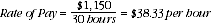1332.0.55.002 - Statistical Language!, 2008
Latest ISSUE Released at 11:30 AM (CANBERRA TIME) 27/06/2008  First Issue
Page tools:Print AllRSSSearch this Product
Contents >> Rate >> How is the Rate calculated?

HOW IS THE RATE CALCULATED?

To calculate the rate of two different units, you divide the units to get a proportion. The denominator is usually the value of most interest. If we return to ‘The Widget Company’, the rate of money earned can be calculated by dividing the pay by number of hours worked.

If we know a manager receives \$1,150 per week and works thirty hours a week, we can work out the amount of pay for each hour worked.A manager working for ‘The Widget Company’ receives \$38.33 per hour worked.

 Previous Page Next Page